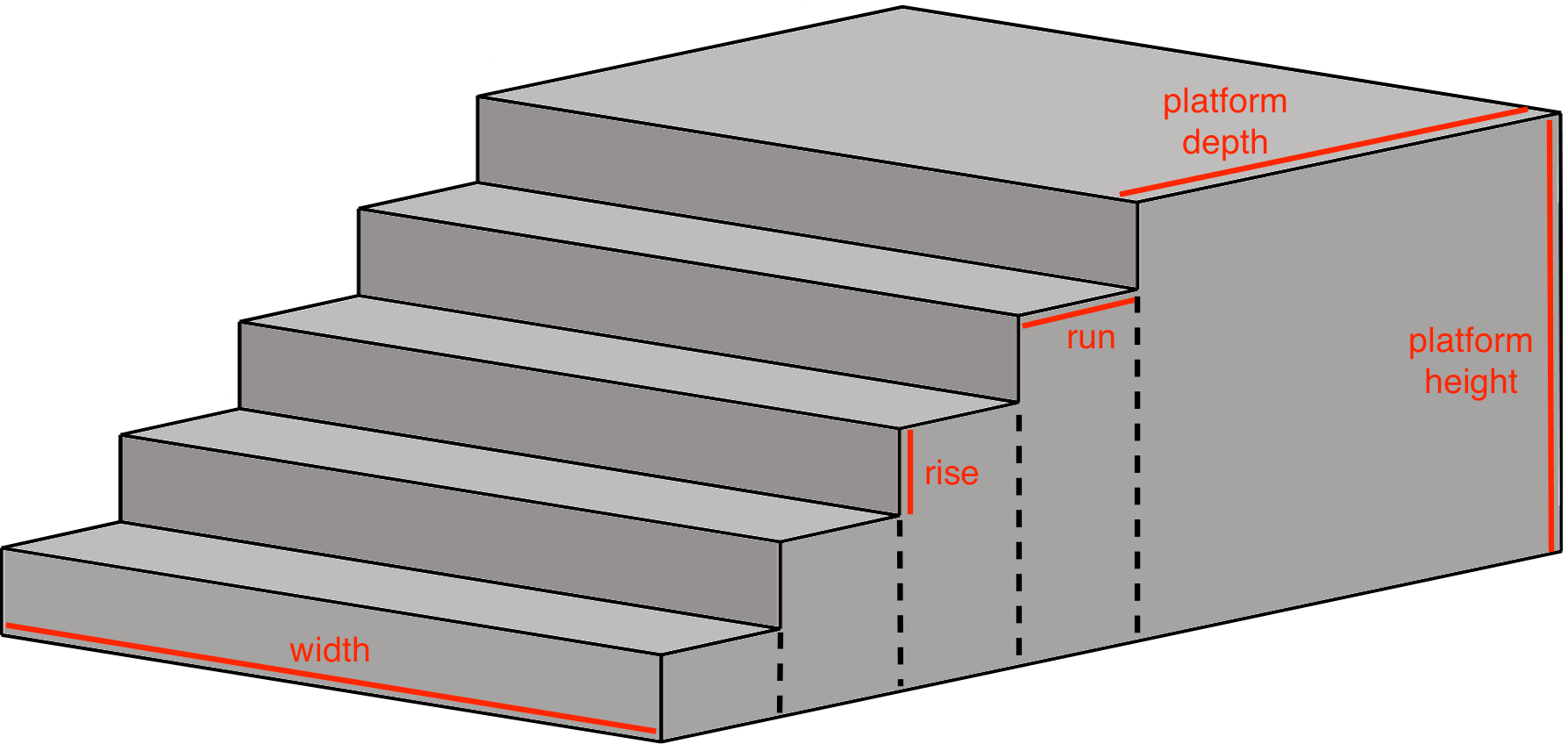# Concrete Steps Calculator

Find the amount of concrete needed to pour steps. The calculator also supports a platform at the top of the steps, leave this empty to use the run dimension for the top step.

Step Dimensions
Optionally specify a custom platform depth for the top step
Optionally enter the price per yd3
\$

## Results:

 Cubic Yards: Cubic Meters:

### Pre-mixed Bags

 50-lb Bags: 60-lb Bags: 80-lb Bags:

### Estimated Concrete Cost

\$
Learn how we calculated this below

## How to Estimate Concrete for Steps

Concrete is a popular material for both short and long runs of steps. It’s durable, long-lasting, and fairly easy to work with. Estimating the concrete needed to pour steps may seem daunting, but because it’s a simple shape to estimate, it can be done in a few simple steps.### Divide the Steps into Rectangles

The first step to estimate the volume of a set of steps is to divide the steps into smaller rectangles. Finding the volume of a rectangular prism is much easier than finding the volume of a complex shape. Think about each step as a rectangle from the top of the step to the ground and the platform (the largest step at the top) as a single rectangle.

You’ll find the volume of each one of these separately, then add them together to find the total volume for the concrete pour.

Find the dimensions of each rectangle and measure the volume for them separately. Use our concrete calculator to assist in this process.

The dimensions of each rectangle will be the run (or depth) by the vertical rise from the ground for that step, multiplied by its width.

For example, if the steps you are pouring are 48″ wide, then the width of each step and the platform will be 48″.

If the depth of each stair tread is 10″ and the depth of the platform itself is 24″, you will then need the height of each step.

The first step’s volume may be:

48″ × 10″ × 8″ = 3,840 cu in

The second step may be:

48″ × 10″ × 16″ = 7,680 cu in

The dimensions of the platform can be found by multiplying the platform depth by the height of the platform from the ground by the width of the total stairs.

### Find the Volume of Each Rectangle Section

The dimensions of each rectangle can be used to find the volume of each using the formula for volume, length × width × height. Simply multiply the step width by the depth and height for each rectangle section.

### Find the Total Step Volume

The final step is to add the volumes of each step section together to get the total volume of the steps. If your measurements are in inches, then. your total volume will be in cubic inches.

Because most concrete is poured in cubic yards, you will need to convert your final measurements.

For example, if you have been measuring the volume for a set of 5 steps with a width of 48″, tread depths of 10″, a platform of 24″ in depth, and a total height of 40″, your final stairs would have a volume of 84,480 cubic inches.

To get cubic yards, you would divide your total cubic inches by 46,660. In this case, you would need 1.81 cubic yards of concrete to complete the pour.

Adding 10% for waste and potential voids gets 1.99 cubic yards, which would most likely be rounded to 2 full yards.

Our concrete step volume calculator can help find how much concrete you need to pour your steps, but you can also hire a professional contractor to measure and pour your steps.